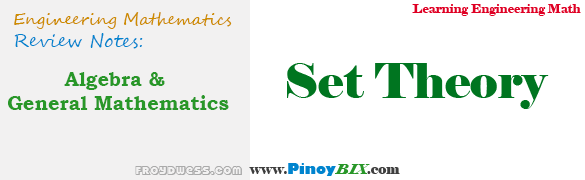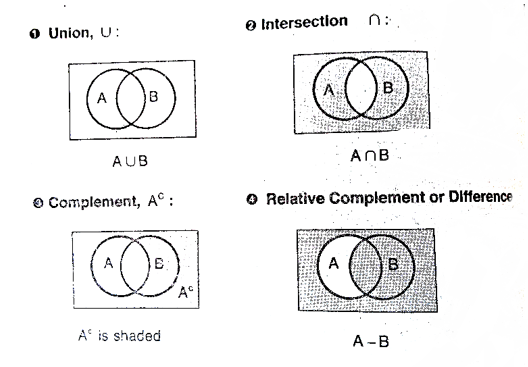# Algebra and General Mathematics: Set Theory

(Last Updated On: February 5, 2020)Set theory is the branch of mathematical logic that studies sets, which informally are collections of objects. The modern study of set theory was initiated by Georg Cantor and Richard Dedekind in the 1870s.

A set is any well defined collection of symbols or objects. The objects comprising the set are called elements or members.

### REPRESENTATION OF A SET

Tabular Form – all the elements of the set are written in braces ( ), each one of them are separated by a comma.

Example:

If set A is a set of counting numbers, then:

A = { 1, 2, 3, 4, 5, 6, …}

Set Builder Form – the elements are not written explicitly but their characteristic properties are stated and written within braces.

Example:

If set A is a set of natural numbers less than 12, then:

A = { x | x is a natural number < 12 }

where:

“|” is read as “such that”

### KINDS OF SETS

Finite Set – a set containing a finite number of elements.

Example:

A = { x | x is a natural number ≤ 6 } or A = { 1, 2, 3, 4, 5, 6 }

Infinite Set – a set containing an infinite number of elements.

Example:

A = { x | x is a natural number } or A = { 1, 2, 3, 4, … }

Singleton – a set having only one element (unit set).

Example:

A = { x | x is a natural number > 5 but < 7 } or A = { 6 }

Null Set – a set having no element. Also called empty set or vid.

Example:

A = { x | x is a natural number between 2 and 3 } or  A = φ

Subset – a set “A” is a subset of another set B, if every element of the set “A” belongs to the set B, denoted by A ⊆ B which is read as “ a is a subset of B” or “A is contained in B”.

Properties of Subset:

• 1. Every set is a subset of itself : A ⊆ A
• 2. Null set is a subset of every set : φ ⊆ A

Proper Subset – a set “A” is said to be a proper subset of a set B if A is a subset of B but is not a subset of A, denoted by A ⊂ B.

Example:

A = { x | x is an odd integer },

B = { x | x is an integer }

Then, A ⊂ B

Universal Set – a set U is called a universal set, if all the sets under discussion are subsets of the set U.

Example:

A = { a, c, e, g,  i, k }

B = {b, d, f, h, j, l, }

C = { a, b, c, d, e, f, g, h, I, j, k, l, … }

Since, set A and B are subset of these set C, the set C is the Universal Set.

Equal Sets – two sets A and B are said to be equal if:

A ⊆ B and B ⊆ A

Example:

A = { 1, 3, 5, 7, 9 } and B = { 1, 3, 5, 7, 9 }

Then, A = B

Equivalent Sets – two sets A and B having equal number of distinct elements are said to be equivalent sets.

Example:

A = { 2, 3, 4, 5, 6, 7 }

B = {a, b, c, d, e, f }

Since, both sets have the same number of distinct elements, the two sets are said to be equivalent sets.

Notes to Remember:

• Equal sets are always equivalent sets but not all equivalent sets are equals
• Infinite sets are always equivalent
• Two sets are equivalent if their Cardinal Number or Order is the same.

### CARDINAL NUMBER OF A SET

The number of distinct elements in a set A is called Cardinal Number or its Order and is denoted by n(A).

Example:

A = { 1, 3, 5, 7, 9, 11, 13 }

n(A) = 7 ; since there are 7 distinct elements

### SET OPERATIONS

Union – the union of sets A and B, denoted by A ⋃ B, is the set of elements which belong to A or B or both A and B. It is one of the fundamental operations through which sets can be combined and related to each other.

Example:

A = { x is an even integer larger than 1 }

B = { x is an odd integer larger than 1 }

A ⋃ B = { 2, 3, 4, 5, 6, … }

Sets cannot have duplicate elements, so the union of the sets { 1, 2, 3 } and { 2, 3, 4 } is { 1, 2, 3, 4 }. Multiple occurrences of identical elements have no effect on the cardinality of a set or its contents.

The number 9 is not contained in the union of the set of prime numbers {2 , 3, 5, 7, 11, …} and the set of even numbers { 2, 4, 6, 8, 10, …}, because 9 is neither prime nor even.

Intersection – the intersection of sets A and B, denoted by A ⋂ B, is the set of elements which belong to both A and B.

Example:

A = { e, f, r, e, n }

B = { k, a, r, e, n }

A ⋂ B = { r, e, n }

If a and B do not have any element in common, that is A ⋂ B = ⌀, then A and B are said to disjoint.

Difference – the difference of sets A and B, is the set of elements which belong to  A but not B.

Example:

A = { e, f, r, e, n }

B = { k, a, r, e, n }

A – B = { e, f } and also, B – A = { k, a }

Complement – the complement of set A, denoted by Ac, is the set of elements which belong to the universal set but not to the set A.

Example:

A = { 1, 3, 5, 7, 9, 11, … } and U = { 1, 2, 3, 4, … }

Ac = { 2, 4, 6, 8, 10, 12, … }

### VENN DIAGRAM – John Venn, (1834 – 1883)

a graphical representation defining set of operations.Sample Problem: ECE Board November 1998

A club of 40 executives, 33 likes to smoke Marlboro and 20 like to smoke Philip Morris. How many like both?

Solution:

Let: x = number of executives who likes to smoke both Marlboro and Philip Morris.

(33 – x ) + (20 – x) + x = 40

x = 53 – 40

x = 13, number of executives who likes to smoke both Marlboro and Philip Morris.

### LAWS OF THE ALGEBRA OF SETS

Idempotent Laws

• A ⋃ A = A
• A ⋂ A = A

Associative Laws

• (A ⋃ B) ⋃ C = A ⋃ (B ⋃ C)
• (A ⋂ B) ⋂ C = (A ⋂ B) ⋂ C

Commutative Laws

• A ⋃ B = B ⋃ A
• A ⋂ B = B ⋂ A

Distributive Laws

• A ⋃ (B ⋂ C) = (A ⋃ B) ⋂ (A ⋃ C)
• A ⋂ (B ⋃ C) = (A ⋂ B) ⋃ (A ⋂ C)

Identity Laws

• A ⋃ ⌀ = A
• A ⋂ ⌀ = A

Complement Laws

• A ⋃ Ac = U
• (Ac)c = A
• A ⋂ Ac = ⌀
• Uc = ⌀, ⌀c = U

De Morgan’s Law

• (A ⋃ B)c = Ac ⋂ Bc
• (A ⋂ B)c = Ac ⋃ Bc
Help Me Makes a Difference!

 P inoyBIX educates thousands of reviewers/students a day in preparation for their board examinations. Also provides professionals with materials for their lectures and practice exams. Help me go forward with the same spirit. “Will you make a small \$5 gift today?” Option 1 : \$1 USD Option 2 : \$3 USD Option 3 : \$5 USD Option 4 : \$10 USD Option 5 : \$25 USD Option 6 : \$50 USD Option 7 : \$100 USD Option 8 : Other Amount© 2014 PinoyBIX Engineering. © 2019 All Rights Reserved | How to Donate? |#### GEAS Solution

Dynamics problem Economics problem Physics problem Statics problem Strength problem Thermodynamics problem

#### Questions and Answers in GEAS

Engineering Economics Engineering Laws and Ethics Engineering Management Engineering Materials Engineering Mechanics General Chemistry Physics Strength of Materials Thermodynamics
Consider Simple Act of Caring!: LIKE MY FB PAGE

Our app is now available on Google Play, Pinoybix Elex